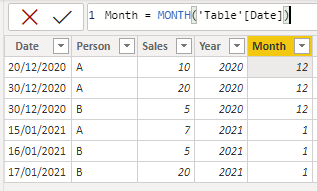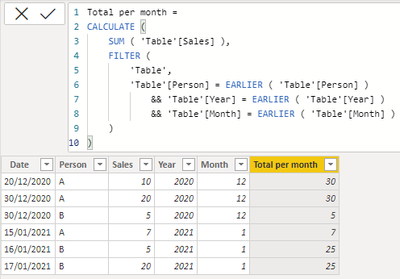cancel
Showing results for
Did you mean:Regular Visitor

## Sum values by category and by month

Hi, I have a dataset where I have data for, let's say, people and their amount of sales. This data is per month and per year. I need to filter the total of their sales by month (of that year) and put this in a new column (Total per month).

 Date Person Sales Total per month 20/12/2020 A \$10 \$30 30/12/2020 A \$20 \$30 30/12/2020 B \$5 \$5 15/01/2021 A \$7 \$7 16/01/2021 B \$5 \$25 17/01/2021 B \$20 \$25

I'm currently working with:

"Total per month = CALCULATE ( SUM ( Table1[Sales] ), ALLEXCEPT( Table1 , Table1[Person] ))"

but this considers sales for every month and year, so what can I add for this to result in the table written above? Thanks in advance.

1 ACCEPTED SOLUTIONSuper User

@actroyani  you can try this

``````Column =
VAR _0 =
ADDCOLUMNS ( tbl, "year", YEAR ( tbl[Date] ) ),
"month", MONTH ( tbl[Date] )
)
VAR _1 =
_0,
"test",
SUMX (
FILTER (
_0,
[Person] = EARLIER ( [Person] )
&& [year] = EARLIER ( [year] )
&& [month] = EARLIER ( [month] )
),
[Sales]
)
)
RETURN
MAXX (
FILTER ( _1, [Person] = EARLIER ( tbl[Person] ) && [Date] = EARLIER ( [Date] ) ),
[test]
)``````Proud to be a Super User!

New Animated Dashboard: Sales Calendar

2 REPLIES 2Community Support

Hi, @actroyani

1. Add month and year to calculation column.

``````Year = YEAR('Table'[Date])

Month = MONTH('Table'[Date])
``````2. Calculate the sum of sales based on the filter of year, month and person.

``````Total per month =
CALCULATE (
SUM ( 'Table'[Sales] ),
FILTER (
'Table',
'Table'[Person] = EARLIER ( 'Table'[Person] )
&& 'Table'[Year] = EARLIER ( 'Table'[Year] )
&& 'Table'[Month] = EARLIER ( 'Table'[Month] )
)
)
``````Best Regards,

Community Support Team _Charlotte

If this post helps, then please consider Accept it as the solution to help the other members find it more quickly.Super User

@actroyani  you can try this

``````Column =
VAR _0 =
ADDCOLUMNS ( tbl, "year", YEAR ( tbl[Date] ) ),
"month", MONTH ( tbl[Date] )
)
VAR _1 =
_0,
"test",
SUMX (
FILTER (
_0,
[Person] = EARLIER ( [Person] )
&& [year] = EARLIER ( [year] )
&& [month] = EARLIER ( [month] )
),
[Sales]
)
)
RETURN
MAXX (
FILTER ( _1, [Person] = EARLIER ( tbl[Person] ) && [Date] = EARLIER ( [Date] ) ),
[test]
)``````Proud to be a Super User!

New Animated Dashboard: Sales Calendar